• Last Updated : 19 Feb, 2021

Python is a great language for doing data analysis, primarily because of the fantastic ecosystem of data-centric Python packages. Pandas is one of those packages and makes importing and analyzing data much easier.

`Dataframe.add()` method is used for addition of dataframe and other, element-wise (binary operator add). Equivalent to dataframe + other, but with support to substitute a fill_value for missing data in one of the inputs.

Parameters:

other :Series, DataFrame, or constant
axis :{0, 1, ‘index’, ‘columns’} For Series input, axis to match Series index on
fill_value : [None or float value, default None] Fill missing (NaN) values with this value. If both DataFrame locations are missing, the result will be missing.
level : [int or name] Broadcast across a level, matching Index values on the passed MultiIndex level

Returns: result DataFrame

 `# Importing Pandas as pd ` `import` `pandas as pd ` ` `  `# Importing numpy as np ` `import` `numpy as np ` ` `  `# Creating a dataframe ` `# Setting the seed value to re-generate the result. ` `np.random.seed(``25``) ` ` `  `df ``=` `pd.DataFrame(np.random.rand(``10``, ``3``), columns ``=``[``'A'``, ``'B'``, ``'C'``]) ` ` `  `# np.random.rand(10, 3) has generated a ` `# random 2-Dimensional array of shape 10 * 3 ` `# which is then converted to a dataframe ` ` `  `df `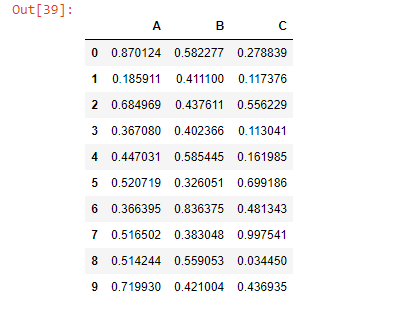Note: `add() `function is similar to ‘+’ operation but, `add()` provides additional support for missing values in one of the inputs.

 `# We want NaN values in dataframe.  ` `# so let's fill the last row with NaN value ` `df.iloc[``-``1``] ``=` `np.nan ` ` `  `df `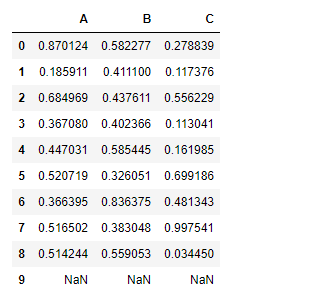Adding a constant value to the dataframe using `add()` function:

 `# add 1 to all the elements ` `# of the data frame ` `df.add(``1``) ` ` `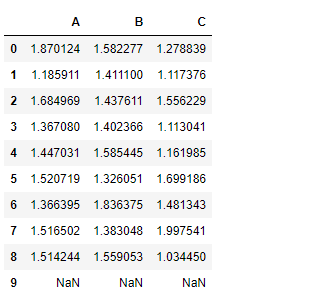Notice the output above, no addition took place for the nan cells in the df `dataframe.add() `function has an attribute `fill_value`. This will fill the missing value(Nan) with the assigned value. If both dataframe values are missing then, the result will be missing.

Let’s see how to do it.

 `# We have given a default value ` `# of '10' for all the nan cells ` `df.add(``1``, fill_value ``=` `10``) `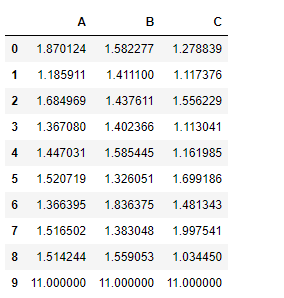All the nan cells has been filled with 10 first and then 1 is added to it.

For Series input, the dimension of the indexes must match for both data frame and series.

 `# Create a Series of 10 values ` `tk ``=` `pd.Series(np.ones(``10``)) ` ` `  `# tk is a Series of 10 elements ` `# all filled with 1 `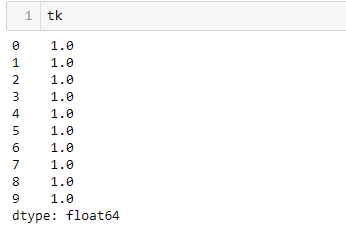`# Add tk(series) to the df(dataframe) ` `# along the index axis ` `df.add(tk, axis ``=``'index'``) `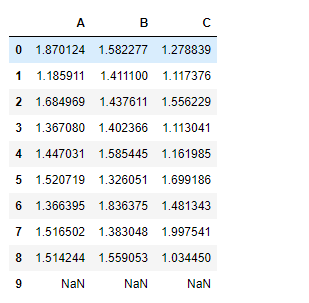Adding one data frame with other data frame

 `# Create a second dataframe ` `# First set the seed to regenerate the result ` `np.random.seed(``10``) ` ` `  `# Create a 5 * 5 dataframe ` `df2 ``=` `pd.DataFrame(np.random.rand(``5``, ``5``), columns ``=``[``'A'``, ``'B'``, ``'C'``, ``'D'``, ``'E'``]) ` ` `  `df2 `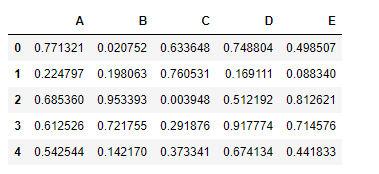Let’s perform element-wise addition of these two data frames

 `df.add(df2) `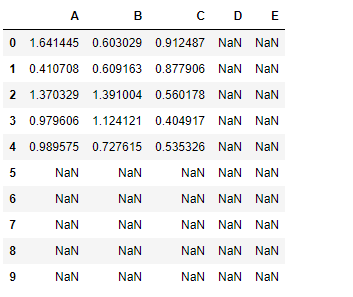Notice the resulting dataframe has dimension 10*5 and it has nan value in all those cells for which either of the dataframe has nan value.

Let’s fix it –

 `# Set a default value of 10 for nan cells ` `# nan value won't be filled for those cells ` `# in which both data frames has nan value ` `df.add(df2, fill_value ``=` `10``) `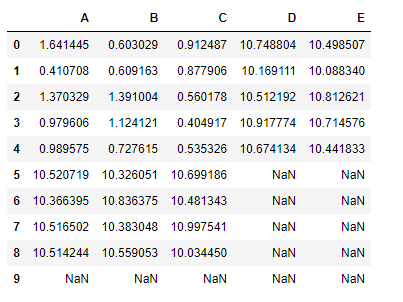My Personal Notes arrow_drop_up
Recommended Articles
Page :